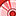# New to Qlik Sense

If you’re new to Qlik Sense, start with this Discussion Board and get up-to-speed quickly.

Announcements
cancel
Showing results for
Did you mean:Contributor

## How to Calculate Percentages based on Different totals?

I need to Calculate Percentages of Male / Females based on certain criteria as listed below under each Lead, I tried  the following but it calculates the % considering the Grand total , I need a formula in Expression to calculate this percentage considering the Total of Male/Females under each Lead rather than the Grand total.

Count({1<[End Date] = {">=\$(=Date(Today(), 'DD/MM/YYYY'))","-"}>}distinct [UID])
/
(Count({1<[End Date] = {">=\$(=Date(Today(), 'DD/MM/YYYY'))","-"}>}distinct TOTAL [UID])-(Count({<[Employee ID] = {"-"}>}distinct TOTAL [UID])))

 Lead Gender Count % of Total Need this Correct % of Total A Male 73 8% 68.22% Female 34 4% 31.78% B Male 9 1% 69.23% Female 4 0% 30.77% C Male 54 6% 79.41% Female 14 2% 20.59% D Male 106 12% 85.48% Female 18 2% 14.52% E Male 336 39% 82.56% Female 71 8% 17.44% F Male 40 5% 75.47% Female 13 1% 24.53% G Male 16 2% 100.00% H Male 20 2% 83.33% Female 4 0% 16.67% I Male 40 5% 72.73% Female 15 2% 27.27% 867 100%
3 RepliesMaster

HI,

Include Lead dimension inside like below

=(Count(distinct ID)/ Count( TOTAL <Lead> DISTINCT ID) ) *100

Count({1<[End Date] = {">=\$(=Date(Today(), 'DD/MM/YYYY'))","-"}>}distinct [UID])
/
(Count({1<[End Date] = {">=\$(=Date(Today(), 'DD/MM/YYYY'))","-"}>}TOTAL <Lead>  distinct  [UID])-(Count({<[Employee ID] = {"-"}>} TOTAL <Lead>  distinct  [UID])))

Thanks,

SasiContributor
Author

Thank you Sasi, but it doesn't seem to be working.Master

Would you be able share some sample data??